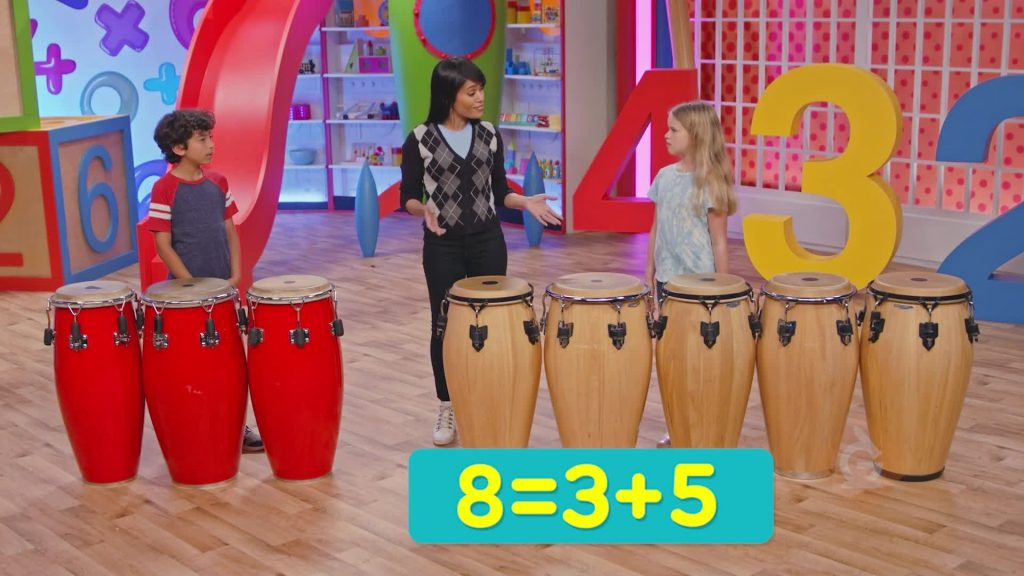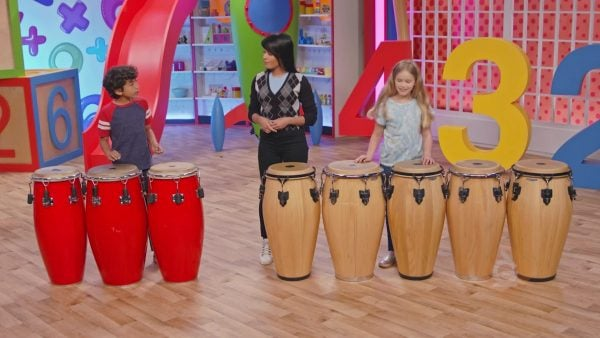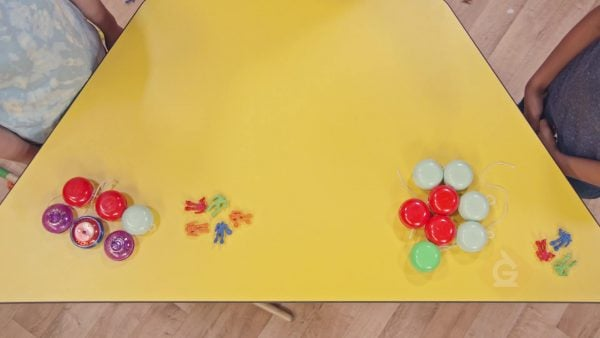Intro to Equality (Find a Missing Number) Math Video | K, 1st & 2nd Grade
1%
It was processed successfully!WHAT IS INTRO TO EQUALITY (FIND A MISSING NUMBER)?

The equals sign “=” tells you that things are equal. When you look at an equation, the equals sign says the amount on the left is the same as the amount on the right.

To better understand intro to equality (finding a missing number)…

WHAT IS INTRO TO EQUALITY (FIND A MISSING NUMBER)?. The equals sign “=” tells you that things are equal. When you look at an equation, the equals sign says the amount on the left is the same as the amount on the right. To better understand intro to equality (finding a missing number)…

## LET’S BREAK IT DOWN!

### Write an equation to show how many Drums.You have 3 drums and 5 drums. That’s 8 drums in all. You can write equations to show the number of drums: 3 + 5 = 8 or 8 = 3 + 5. The equal sign means that the amounts on both sides are the same. 8 is equal to 8.

Write an equation to show how many Drums. You have 3 drums and 5 drums. That’s 8 drums in all. You can write equations to show the number of drums: 3 + 5 = 8 or 8 = 3 + 5. The equal sign means that the amounts on both sides are the same. 8 is equal to 8.

### Write an equation with a missing number.There are 6 muffins and some more muffins. There are 15 muffins in all. You can use an equation to show how many muffins. 15 = 6 + ? or 6 + ? = 15. The ? shows that there is something you don’t know.

Write an equation with a missing number. There are 6 muffins and some more muffins. There are 15 muffins in all. You can use an equation to show how many muffins. 15 = 6 + ? or 6 + ? = 15. The ? shows that there is something you don’t know.

### Write an equation to find the missing number of muffins.You know that 15 = 6 + ?. What is the missing number? Count on from 6 to 15: 7, 8, 9, 10, 11, 12, 13, 14, 15. You counted on 9 in all. 15 = 6 + 9. The missing number of muffins is 9.

Write an equation to find the missing number of muffins. You know that 15 = 6 + ?. What is the missing number? Count on from 6 to 15: 7, 8, 9, 10, 11, 12, 13, 14, 15. You counted on 9 in all. 15 = 6 + 9. The missing number of muffins is 9.

### Write an equation to find how many birds flew away.A nest has 12 baby birds. You saw some birds fly away. Now there are 5 baby birds in the nest. How many flew away? 12 – ? = 5. You know that 5 + 7 = 12, so the missing number must be 7. 7 birds flew away.

Write an equation to find how many birds flew away. A nest has 12 baby birds. You saw some birds fly away. Now there are 5 baby birds in the nest. How many flew away? 12 – ? = 5. You know that 5 + 7 = 12, so the missing number must be 7. 7 birds flew away.

### Party FavorsYou won 5 cards and 6 yo-yos. That is 5 + 6 prizes in all. Your friend won 3 cards and 8 yo-yos. That’s 3 + 8 prizes in all. 5 + 6 = 11. 3 + 8 = 11. So, 5 + 6 = 3 + 8. You and your friend won the same number of prizes.

Party Favors You won 5 cards and 6 yo-yos. That is 5 + 6 prizes in all. Your friend won 3 cards and 8 yo-yos. That’s 3 + 8 prizes in all. 5 + 6 = 11. 3 + 8 = 11. So, 5 + 6 = 3 + 8. You and your friend won the same number of prizes.

## INTRO TO EQUALITY (FIND A MISSING NUMBER) VOCABULARY

Equal sign
Means that the amounts on both sides of it are the same.
Equation
Says that two things are equal. 6 + 3 = 9.
Total
The whole amount.
True equation
Values on each side of the equal sign are the same.
False equation
Values on each side of the equal sign are not the same.
Equal
Exactly the same amount or value.
An equation may show that one part is unknown. You may see it as ____ or ?, for example, 3 + ? = 10.

## INTRO TO EQUALITY (FIND A MISSING NUMBER) DISCUSSION QUESTIONS

4 + 9 = 13.

9, 8 + 9 = 17.

8; 6 = 14 – 8

7; 7 – 3 = 4

### Nancy has 6 green apples and 7 red apples. Padma has 9 red apples and 4 green apples. Do Nancy and Padma have the same number of apples? How do you know?

Nancy has 6 + 7, or 13 apples. Padma has 9 + 4, or 13 apples. 6 + 7 = 13 and 9 + 4 = 6 + 7 and 13 = 13. Nancy and Padma each have 13 apples.
X

## Success

We’ve sent you an email with instructions how to reset your password.
Ok